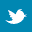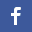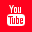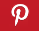Completely Solved C, C++ Programs Assignment.Quick Search Database Videos Tutorials Ebooks Forums FAQ Aboutus Household Industrial Manufacturing Service Shopping Transportation### C program to implement Floyd's algorithm which will produce shortest distance between all vertex pairs of a weighted graph.

 Filed Under: C Assignments

Program Statement :
Write a C program to implement Floyd's algorithm which will produce shortest distance between all vertex pairs of a weighted graph.

Theory :
Floyd's algorithm is one of the shortest path algorithms in graph theory. A weighted graph is taken as input in the form of weight-matrix. Weight-matrix is a vertex by vertex matrix where, w(i,j) = weight between vertices vi and vj. If there is no edge between vi and vj then w(i,j) = Infinity (a very large number i.e 9999 used as input for giving infinity in the actual program). Diagonal elements are always zero for a simple graph. The output of the algorithm is also a matrix of same order as the input, where we can find shortest distance between all vertex pairs. Three for loops are used in the program and total number of iterations are same as the number of vertices of the graph. For example - if number of vertices are 5 then we get the output matrix after 5th iteration. At iteration k, the algorithm must check for each pair of node(i,j) whether or not there exsists a path from i to j passing through the node k that is greater than the present optimal path.

Algorithm :
Algo_floyd(w[size][size], n) /*The input graph and no. of vertices n passed as parameters*/
{
For(k=1 to n) /*k represents table no.*/
{
For(i=1 to n) /*i represents row no. within a table*/
{
For(j=1 to n) /*j represents column no. within a row*/
{
If(w[i][j] > (w[i][k] + w[k][j])) /*Minimum is to be selected*/
/*w[i][j] denotes distance between ith vertex vi and jth vertex vj*/
w[i][j]=(w[i][k]+w[k][j]);
}
}
}
}
/*End of Algo_floyd*/

Program listing :
/*C program to implement Floyd's algorithm which will produce shortest distance between all vertex pairs */
#include<stdio.h>
#define size 10/*Defining maximum size of the weight-matrix*/
main()
{
int a[size][size];
int i,j,n;
void floyd(int[][j],int);/*Function declaration*/
printf("Enter no. of vertices : ");/*No. of vertex should be less equal to defined size*/
scanf("%d",&n);
printf("Give the initial weighted graph in weight matrix form(give 9999 in the place of infinity) : n");
for(i=0;i<n;i++)
{
for(j=0;j<n;j++)
{
printf("Enter the value of a[%d][%d]:",i,j);/*Giving inputs one by one row-wise,give a 9999 as infinity*/
scanf("%d",&a[i][j]);
}
}
printf("The input weight matrix is:-n");
for(i=0;i<n;i++)
{
for(j=0;j<n;j++)
{
if(a[i][j]==9999)
printf("inft");/*Printing inf as infinity in the input matrix*/
else
printf("%dt",a[i][j]);/*If data is not infinity then print the weight*/
}
printf("n");
}
floyd(a,n);/*Function call*/
printf("nThe final matrix where we can find the shortest distance:n");
for(i=0;i<n;i++)
{
for(j=0;j<n;j++)
printf("%5d",a[i][j]);
printf("n");
}
}
void floyd(int a[size][size],int n)/*Function definition*/
{
int k,i,j;
for(k=0;k<n;k++)/*n is the no.of vertices of the graph and k represents table no.*/
{
for(i=0;i<n;i++)/*i represents row no. within a table*/
{
for(j=0;j<n;j++)/*j represents column no. within a row*/
{
if(a[i][j]>(a[i][k]+a[k][j]))/*Minimum is to be selected*/
/*a[i][j] denotes distance between ith vertex and jth vertex*/
a[i][j]=(a[i][k]+a[k][j]);
}
}
}
}

Output :
Enter no. of vertices : 5
Give the initial weighted graph in weight matrix form(give 9999 in the place of infinity) :

Enter the value of a:0
Enter the value of a:2
Enter the value of a:1
Enter the value of a:5
Enter the value of a:9999
Enter the value of a:2
Enter the value of a:0
Enter the value of a:9999
Enter the value of a:1
Enter the value of a:3
Enter the value of a:1
Enter the value of a:9999
Enter the value of a:0
Enter the value of a:1
Enter the value of a:9999
Enter the value of a:5
Enter the value of a:1
Enter the value of a:1
Enter the value of a:0
Enter the value of a:2
Enter the value of a:9999
Enter the value of a:3
Enter the value of a:9999
Enter the value of a:2
Enter the value of a:0

The input weight matrix is:-
0 2 1 5 inf
2 0 inf 1 3
1 inf 0 1 inf
5 1 1 0 2
inf 3 inf 2 0

The final matrix where we can find the shortest distance:
0 2 1 2 4
2 0 2 1 3
1 2 0 1 3
2 1 1 0 2
4 3 3 2 0

Discussions :
● Number of vertices of the graph must be less than the defined size (or dimension) of the input weight-matrix.
● Weights of edges of the graph given as input must be non-negative real numbers otherwise it will not produce desirable result.
● The complexity of this algorithm is O(n3) and can be solved in polynomial time.
● The infinity value is taken as 9999 as input.Back to main directory:Software Practical